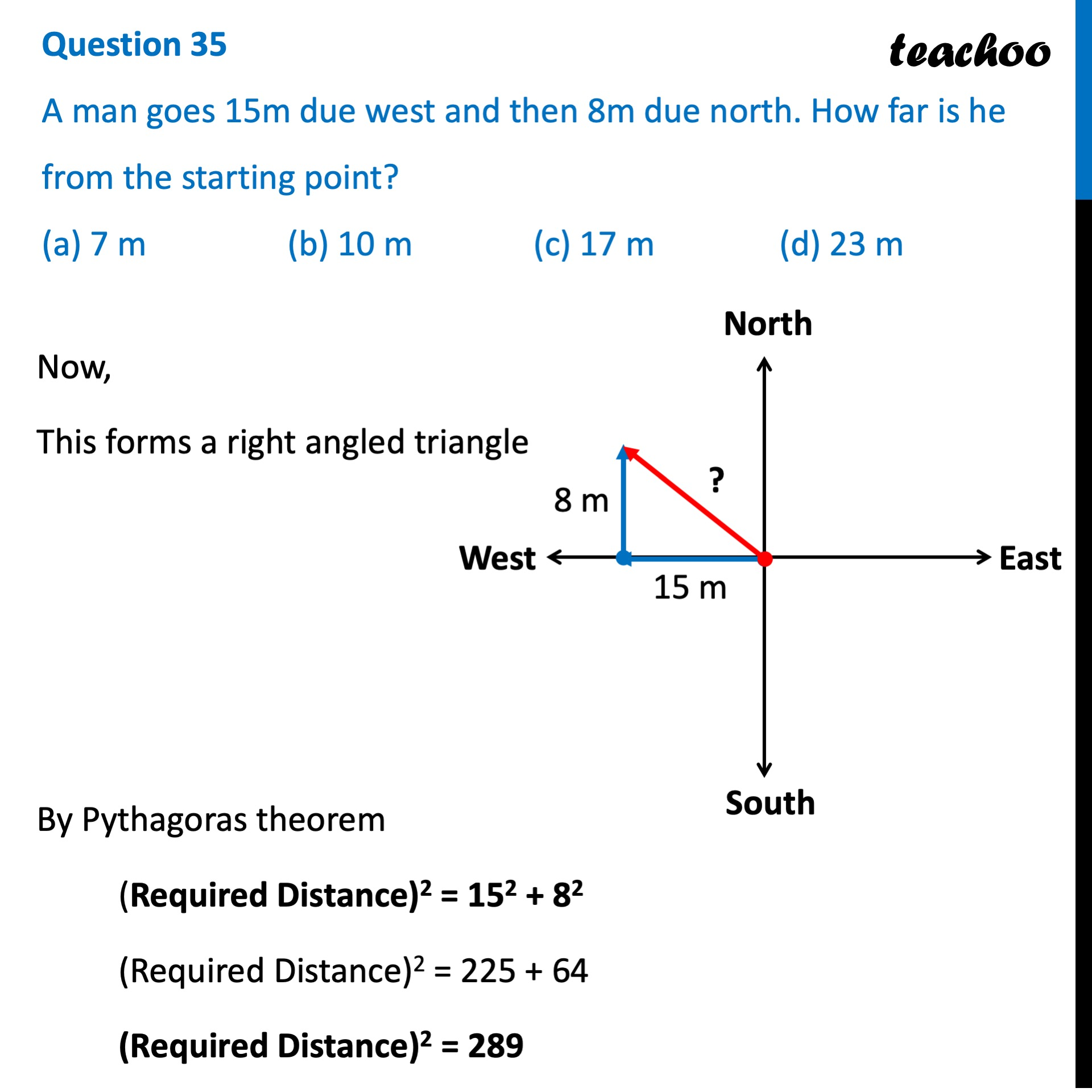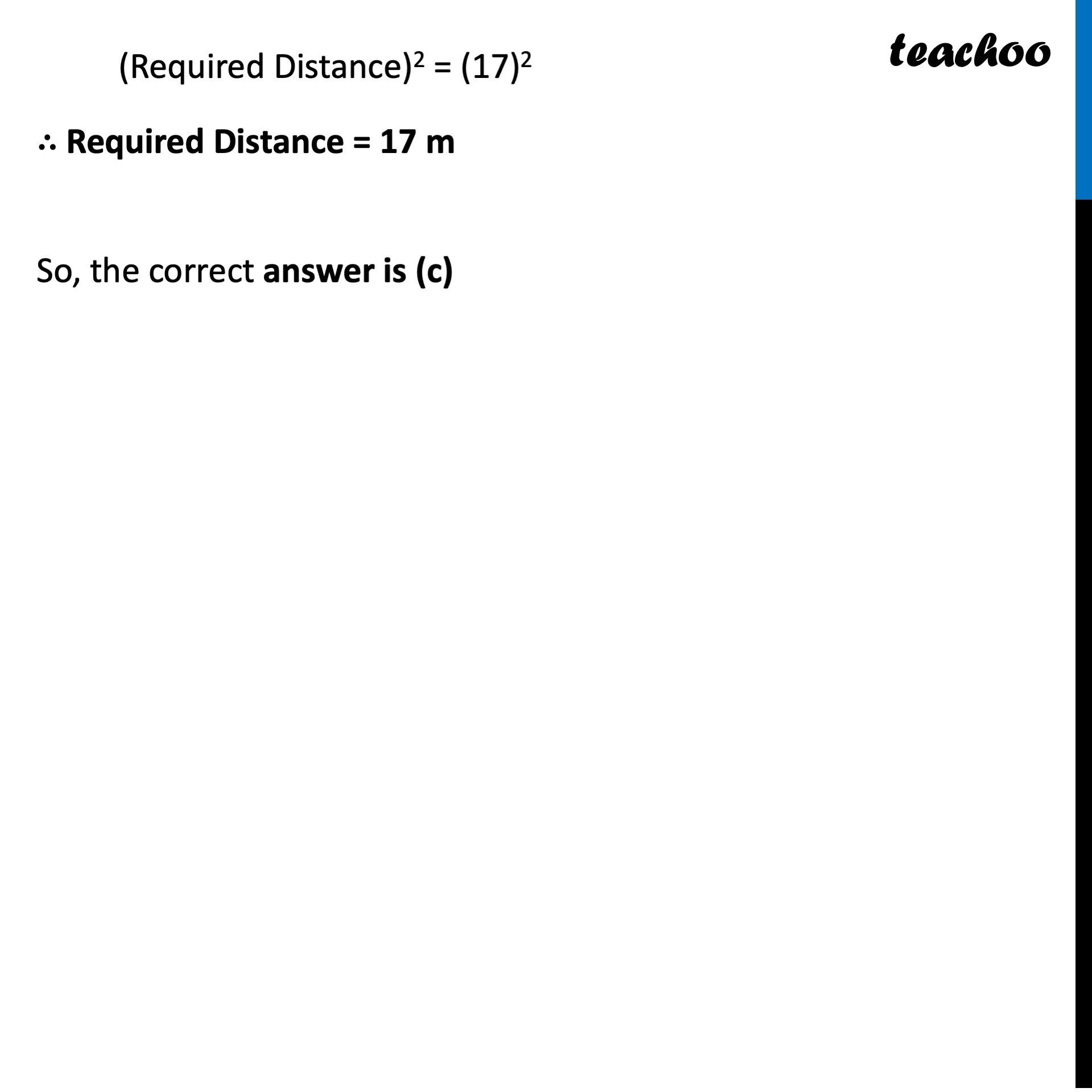CBSE Class 10 Sample Paper for 2022 Boards - Maths Basic [MCQ]

Class 10
Solutions of Sample Papers for Class 10 Boards

### (a) 7 m   (b) 10 m   (c) 17 m   (d) 23 mLearn in your speed, with individual attention - Teachoo Maths 1-on-1 Class

### Transcript

Question 35 A man goes 15m due west and then 8m due north. How far is he from the starting point? (a) 7 m (b) 10 m (c) 17 m (d) 23 m Now, This forms a right angled triangle By Pythagoras theorem (Required Distance)2 = 152 + 82 (Required Distance)2 = 225 + 64 (Required Distance)2 = 289 (Required Distance)2 = (17)2 ∴ Required Distance = 17 m So, the correct answer is (c)